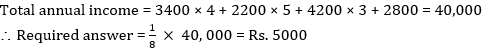# Quantitative MCQ - 8

## 30 Questions MCQ Test Quantitative Aptitude for Competitive Examinations | Quantitative MCQ - 8

Description
This mock test of Quantitative MCQ - 8 for Quant helps you for every Quant entrance exam. This contains 30 Multiple Choice Questions for Quant Quantitative MCQ - 8 (mcq) to study with solutions a complete question bank. The solved questions answers in this Quantitative MCQ - 8 quiz give you a good mix of easy questions and tough questions. Quant students definitely take this Quantitative MCQ - 8 exercise for a better result in the exam. You can find other Quantitative MCQ - 8 extra questions, long questions & short questions for Quant on EduRev as well by searching above.
QUESTION: 1

### A school team has 9 volleyball players. A 6 member team and a captain will be selected out of these nine players. How many different selections can be made?

Solution: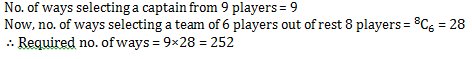QUESTION: 2

### Directions(2-5): What should come in place of question mark(?) in the questions given below?(you are not expected to get exact values). 4859.008 + 274.89 × 24.95 = ?

Solution: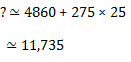QUESTION: 3

### Directions(2-5): What should come in place of question mark(?) in the questions given below?(you are not expected to get exact values). 7534.98 ÷ 501.99 × 1.006 = ?

Solution: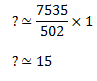QUESTION: 4

Directions(2-5): What should come in place of question mark(?) in the questions given below?(you are not expected to get exact values).

59.89% of 7085.01 – 4/11 of 1077.97 = ?

Solution: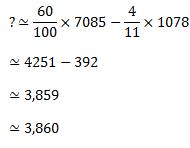QUESTION: 5

Directions(2-5): What should come in place of question mark(?) in the questions given below?(you are not expected to get exact values).

231 + 14.9 × 27.9 + 39.88= ?

Solution: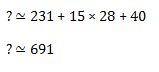QUESTION: 6

Mr. Amit Roy, the renowned author, recently got his new novel released. To his utter dismay he found that for the 850 pages on an average there were 3 mistakes every page. While, in the first  350 pages there were only 550 mistakes, they seemed to increase for the latter pages. Find the average number of mistakes per page for the remaining pages.

Solution: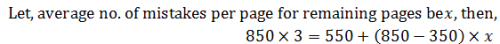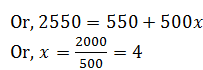QUESTION: 7

There are two mixtures of honey and water, the quantity of honey in them being 40% and 75% of the mixture. If 5 gallons of the first are mixed with 8 gallons of the second, what will be the ratio of honey to water in the new mixture ?

Solution: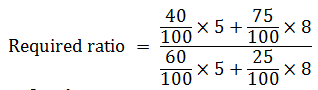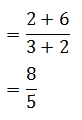QUESTION: 8

A and B have some guavas divided between themselves. A says to B “If I give you 20% of the guavas I have, I will still have 20 more guavas than you have.” To this, B says “If you give me guavas equal to 75% of what I have now, I will have 30 more guavas than you have.” What is the total number of guavas that they have ?

Solution: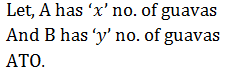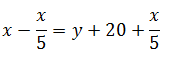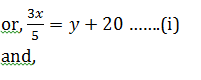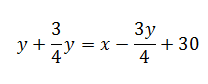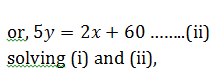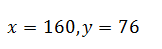QUESTION: 9

A manufacturer sells a pair of glasses to a wholesale dealer at a profit of 17%. The wholesaler sells the same to a retailer at a profit of 25%. The retailer in turn sells them to a customer for Rs. 32.76, thereby earning a profit of 40%. The cost price for the manufacturer is

Solution: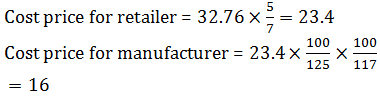QUESTION: 10

Rotomac produces very fine quality of writing pens. Company knows that on an average 20% of the produced pens are always defective so are rejected before packing. Company promises to deliver 7500 pens to its wholesaler at Rs. 15 each. It estimates the overall profit on all the manufactured pens to be 25%. What is the manufacturing cost of each pen ?

Solution: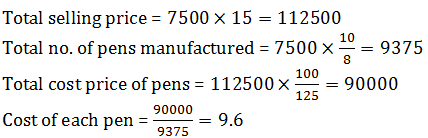QUESTION: 11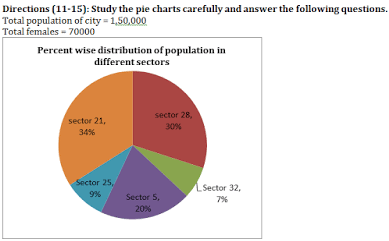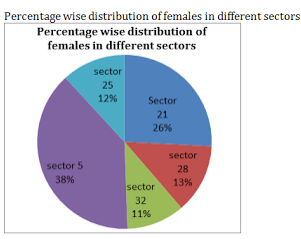What is the total  no. of men in sectors 21,25 and 5 together ?

Solution: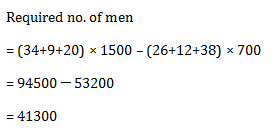QUESTION: 12Total no. of females in sectors 32 and 28 are approximately what percent of total no. of men in these sectors together ?

Solution: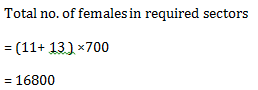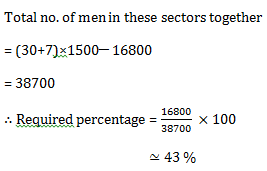QUESTION: 13

No. of men in sector 28 is approximately what percent of the no. of total persons in sector 25?

Solution: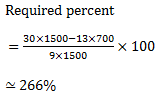QUESTION: 14What is the ratio of no. of males in sector 32 to the no. of females in sector 25?

Solution: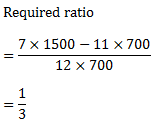QUESTION: 15If 70% persons are eligible for voting from sectors 5 and 21 together and 75% females are eligible for voting from these two sectors together then find the no. of men who are eligible for voting from these two sectors together.

Solution: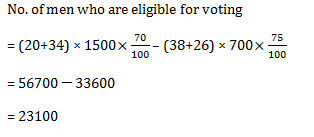QUESTION: 16

David invested certain amount in three different schemes A, B and C with the rate of interest 10% p.a., 12% p.a. and 15% p.a. respectively. If the total interest accrued in one year was Rs. 3200 and the amount invested in Scheme C was 150% of the amount invested in Scheme A and 240% of the amount invested in Scheme B, What was the amount invested in Scheme B?

Solution: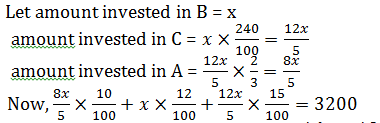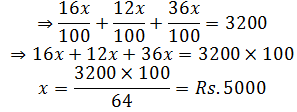QUESTION: 17

A person lent out a certain sum on simple interest and the same sum on compound interest at a certain rate of interest per annum. He noticed that the ratio between the difference of compound interest and simple interest of 3 years and that of 2 years is 25 : 8. The rate of interest per annum is:

Solution: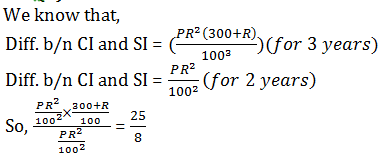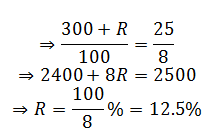QUESTION: 18

A slab of ice 8 inches in length, 11 inches in breadth, and 2 inches thick was melted and resolidified in the form of a rod of 8 inches diameter. The length of such a rod, in inches, is

Solution: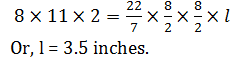QUESTION: 19

Six pipes are fitted to a water tank. Some of these are inlet pipes and the others outlet pipes. Each inlet pipe can fill the tank in 9 hrs and each outlet pipe can empty the tank in 6 hrs.On opening all the pipes, an empty tank is filled in 9 hrs. How many inlet pipes are there?

Solution: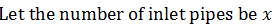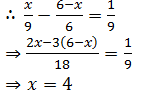QUESTION: 20

In how many different ways can the letters of the word ‘HAPPYIER’ be arranged?

Solution: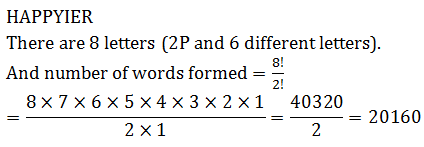QUESTION: 21

Direction for (21 to 23)The following table show the total number of vacancies in five different companies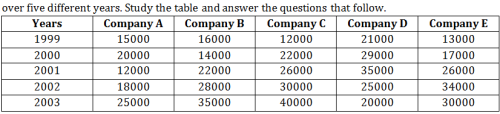What is the average no. of vacancies of in company B over all the years

Solution: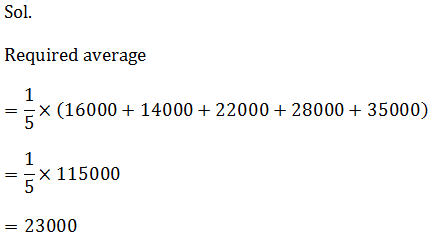QUESTION: 22

Direction for (21 to 23)The following table show the total number of vacancies in five different companiesIn which year, total vacancies are maximum from all companies together?

Solution: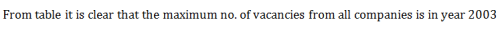QUESTION: 23

Direction for (21 to 23)The following table show the total number of vacancies in five different companiesWhat is the ratio of vacancies in companies B and C together in year 2001 to the no. of vacancies in companies A and D together in year 1999?

Solution: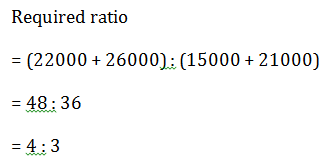QUESTION: 24

Directions (24-27): Study the following graph and answer the questions accordingly:
The following pie-chart shows the percentage distribution of  questions asked from different sections of quant in 10 years in various banking exams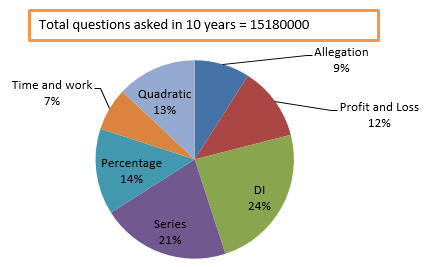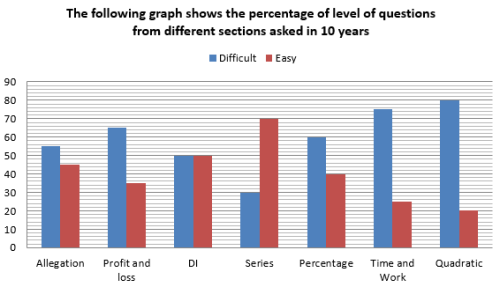The number of difficult questions asked from Allegation section are approximately what percent of  number of difficult questions asked from Quadratic section ?

Solution: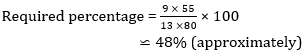QUESTION: 25

Directions (24-27): Study the following graph and answer the questions accordingly:
The following pie-chart shows the percentage distribution of  questions asked from different sections of quant in 10 years in various banking examsWhat is the ratio of total number of easy questions asked from Allegation and Profit and Loss sections together to the total number of easy questions asked from Percentage and Time and work sections together ?

Solution: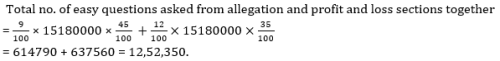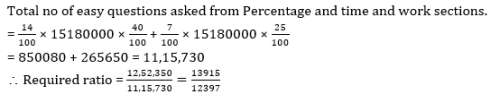QUESTION: 26

Directions (24-27): Study the following graph and answer the questions accordingly:
The following pie-chart shows the percentage distribution of  questions asked from different sections of quant in 10 years in various banking examsThe number of difficult questions asked from Series section are what percent more or less than the total number of questions asked from Percentage section ?

Solution: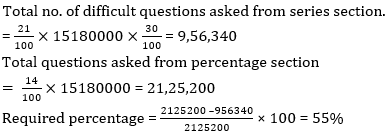QUESTION: 27

Directions (24-27): Study the following graph and answer the questions accordingly:
The following pie-chart shows the percentage distribution of  questions asked from different sections of quant in 10 years in various banking examsWhat is the average number of difficult questions asked from DI,Quadratic and Allegation  sections together ?

Solution: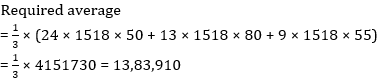QUESTION: 28

Out of three numbers, the first is four times the second and is one third of the third. If the average of the three numbers is 221, what will be the difference between the highest no. and the lowest one?

Solution: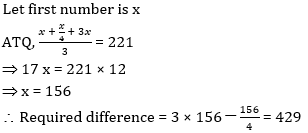QUESTION: 29

The avg. weight of three men A,B and C is 84 kg. Another man D joins the group and the average now becomes 80 kg. If another man E, whose weight is 3 kg more than that of D, replaces A, then the average weight of B,C,D and E becomes 79 kg. What is the weight of A?

Solution: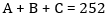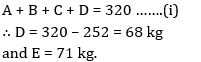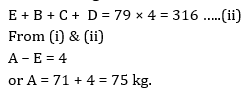QUESTION: 30

The avg. monthly expenditure of a family was Rs. 3400  during the first four months; Rs. 2200 during the next five months and Rs. 4200 during the last three months of a year. If the total savings during the year were Rs. 2800, what was the one eighth of annual income?

Solution: